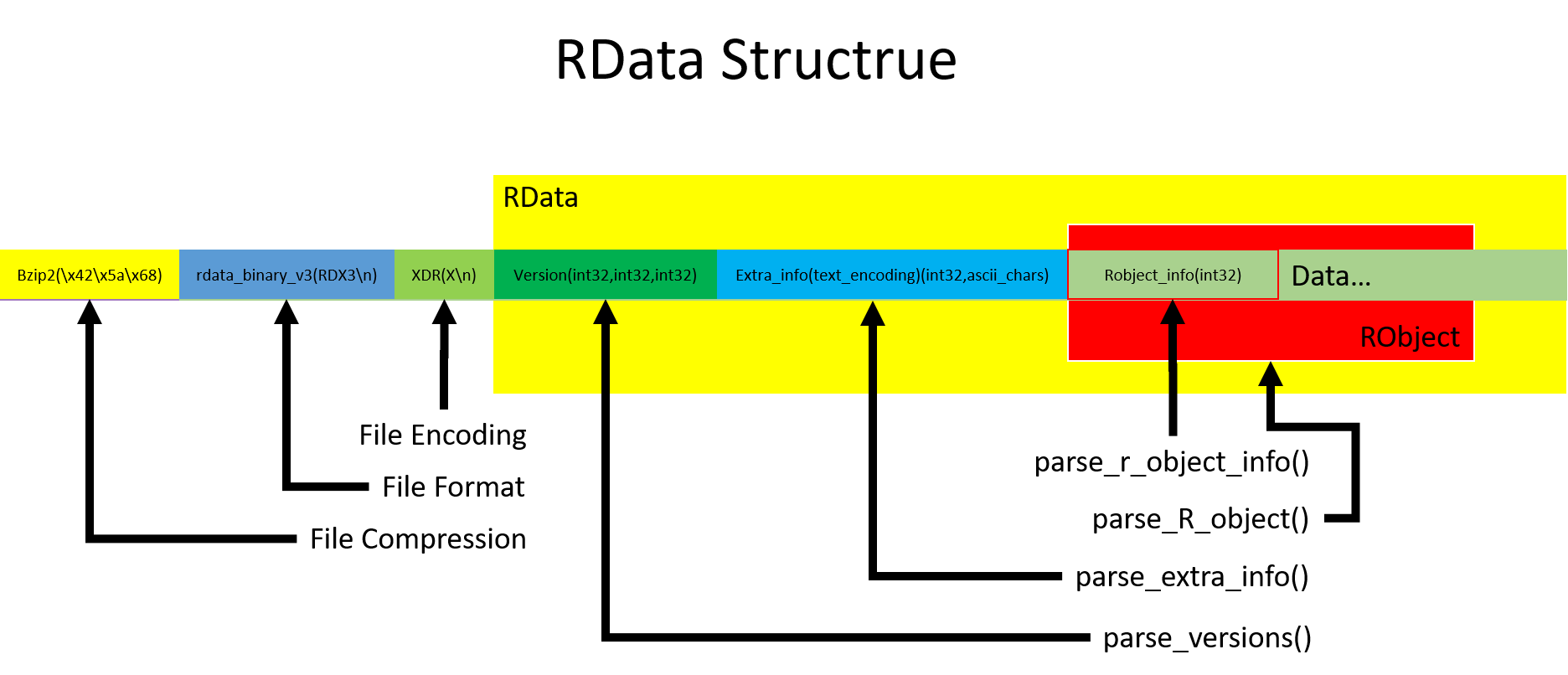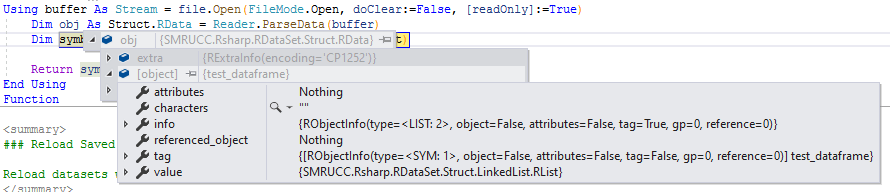## RData文件格式

rdata怎么读取?### RData数据编码基础：XDR编码

XDR编码方案是 1987 年 6 月由 Sun Microsystems，Inc. 编写的 RFC 1014中描述的外部数据表示标准（External Data Representation）。XDR提供了一种与体系结构无关的表示数据，解决了数据字节排序的差异、数据字节大小、数据表示和数据对准的方式。使用XDR的应用程序，可以在异构硬件系统上交换数据。

''' <summary>
''' Decodes the Int32.
''' http://tools.ietf.org/html/rfc4506#section-4.1
''' </summary>
Public Function DecodeInt32(r As IByteReader) As Integer
If r.EndOfStream Then
' Return 0
Throw New InvalidProgramException
Else
' 20211203
' default in VB.NET is byte shift
' should be convert to integer at first
Dim H18 = CInt(r.Read) << &H18
Dim H10 = CInt(r.Read) << &H10
Dim H8 = CInt(r.Read) << &H8

Return H18 Or H10 Or H8 Or H0
End If
End Function

''' <summary>
''' Decodes the Double.
''' http://tools.ietf.org/html/rfc4506#section-4.7
''' </summary>
Public Function DecodeDouble(r As IByteReader) As Double
Dim num As Long = Xdr.XdrEncoding.DecodeInt64(r)
Return unsafeDouble(num)
End Function

''' <summary>
''' Decodes the Int64.
''' http://tools.ietf.org/html/rfc4506#section-4.5
''' </summary>
Public Function DecodeInt64(r As IByteReader) As Long
End Function

Private Function unsafeDouble(x As Long) As Double
Dim bytes = BitConverter.GetBytes(x)
Dim dbl As Double = BitConverter.ToDouble(bytes, 0)

Return dbl
End Function

## 在VisualBasic中解析RData数据集

### 文件级别的解析代码

ReadOnly magic_dict As New Dictionary(Of FileTypes, Byte()) From {
{FileTypes.bzip2, bytes("\x42\x5a\x68")},
{FileTypes.gzip, bytes("\x1f\x8b")},
{FileTypes.xz, bytes("\xFD7zXZ\x00")},
{FileTypes.rdata_binary_v2, bytes("RDX2\n")},
{FileTypes.rdata_binary_v3, bytes("RDX3\n")}
}

ReadOnly format_dict As New Dictionary(Of RdataFormats, Byte()) From {
{RdataFormats.XDR, bytes("X\n")},
{RdataFormats.ASCII, bytes("A\n")},
{RdataFormats.binary, bytes("B\n")}
}

### 文件元数据读取

''' <summary>
''' </summary>
''' <returns></returns>
Public Function parse_versions() As RVersions
Dim format_version = parse_int()
Dim r_version = parse_int()
Dim minimum_r_version = parse_int()

Static supportedVer As New Index(Of Integer) From {2, 3}

If Not format_version Like supportedVer Then
Throw New NotImplementedException(\$"Format version {format_version} unsupported")
End If

Return New RVersions With {
.format = format_version,
.serialized = r_version,
.minimum = minimum_r_version
}
End Function

''' <summary>
''' </summary>
''' <param name="versions"></param>
''' <returns></returns>
Public Function parse_extra_info(versions As RVersions) As RExtraInfo
Dim encoding As String = Nothing
Dim encoding_len As Integer

If versions.format >= 3 Then
encoding_len = parse_int()
encoding = parse_string(encoding_len).decode(Encodings.ASCII)
End If

Dim extract_info = New RExtraInfo With {.encoding = encoding}

Return extract_info
End Function

### 解析RObject对象

''' <summary>
''' Representation of a R object.
''' </summary>
Public Class RObject

Public Property info As RObjectInfo
Public Property value As RList
Public Property attributes As RObject
Public Property tag As RObject
Public Property referenced_object As RObject

End Class

Public Class RList
Public Property CAR As RObject
Public Property CDR As RObject
Public Property data As Array
End Class

''' <summary>
''' Internal attributes of a R object.
''' </summary>
Public Class RObjectInfo

Public type As RObjectType
Public [object] As Boolean
Public attributes As Boolean
Public tag As Boolean
Public gp As Integer
Public reference As Integer

End Class

• CAR为当前的符号对象的值存储链，读取当前的符号值所有的数据都可以通过读取CAR路径来实现
• CDR则链接到了下一个符号对象的数据存储链，如果要读取其他的符号数据，则必须要通过CDR路径来实现

#### RObject元数据读取

''' <summary>
''' Parse the internal information of an object.
''' </summary>
''' <param name="info_int"></param>
''' <returns></returns>
Public Function parse_r_object_info(info_int As Integer) As RObjectInfo
Dim type_exp As RObjectType = bits(info_int, 0, 8)
Dim reference = 0
Dim object_flag As Boolean
Dim attributes As Boolean
Dim tag As Boolean
Dim gp As Integer

If is_special_r_object_type(type_exp) Then
object_flag = False
attributes = False
tag = False
gp = 0
Else
object_flag = CBool(bits(info_int, 8, 9))
attributes = CBool(bits(info_int, 9, 10))
tag = CBool(bits(info_int, 10, 11))
gp = bits(info_int, 12, 28)
End If

If type_exp = RObjectType.REF Then
reference = bits(info_int, 8, 32)
End If

Return New RObjectInfo With {
.type = type_exp,
.[object] = object_flag,
.attributes = attributes,
.tag = tag,
.gp = gp,
.reference = reference
}
End Function

#### RObject对象值类型

' r object
NIL = 0, SYM = 1, LIST = 2, CLO = 3, ENV = 4, PROM = 5, LANG = 6, SPECIAL = 7, BUILTIN = 8
' element vector
CHAR = 9, LGL = 10, INT = 13, REAL = 14, CPLX = 15, STR = 16
' r language
DOT = 17, ANY = 18, VEC = 19, EXPR = 20, BCODE = 21, EXTPTR = 22, WEAKREF = 23, RAW = 24, S4 = 25
' alternative flags
ALTREP = 238, EMPTYENV = 242, GLOBALENV = 253, NILVALUE = 254, REF = 255

#### 链表结构的读取

' parse_R_object
tag = Nothing

If info.attributes Then
attributes = parse_R_object(reference_list)
ElseIf info.tag Then
tag = parse_R_object(reference_list)
End If

Dim car As RObject = parse_R_object(reference_list)
Dim cdr As RObject = parse_R_object(reference_list)

value = New RList With {.CAR = car, .CDR = cdr}

#### 向量的读取

Private Function parseVector(Of T)(parse As Func(Of T)) As Array
Dim length As Integer = parse_int()
Dim value As T() = New T(length - 1) {}

For i As Integer = 0 To length - 1
value(i) = parse()
Next

Return value
End Function

If info.type = RObjectType.LGL Then
ElseIf info.type = RObjectType.INT Then
ElseIf info.type = RObjectType.REAL Then
ElseIf info.type = RObjectType.CPLX Then
ElseIf info.type Like objType3 Then
value = parseVector(Function() parse_R_object(reference_list))

''' <summary>
''' Parse all the file.
''' </summary>
''' <returns></returns>
Public Function parse_all() As RData
Dim versions As RVersions = parse_versions()
Dim extra_info As RExtraInfo = parse_extra_info(versions)
Dim obj As RObject = parse_R_object()

Return New RData With {
.versions = versions,
.extra = extra_info,
.[Object] = obj
}
End Function

## VisualBasic代码用例

Imports SMRUCC.Rsharp.RDataSet

Using buffer As Stream = file.Open(FileMode.Open, doClear:=False, [readOnly]:=True)
Dim obj As Struct.RData = Reader.ParseData(buffer)
' blabla
End UsingLatest posts by 谢桂纲 (see all)

### Order by Date Name Attachments

#### One response

1. […] 从上一篇博客文章之中我们比较下详细的了解了RData数据文件的文件格式以及对应的读取操作。在这篇文章之中我们来了解如何基于我们通过对RData文件读取操作所获取得到的链表数据进行反序列化操作，将R环境之中的数据集串流加载到下游的R#数据分析环境之中。 […]

来自中国
1.2.3.4.空间Spot结果在下载到的mzkit文件夹中有示例吗？我试了试，不是10X结果中的tissue_positions_list.csv（软件选择此文件，直接error）；在默认结果中也没找到类似的文件；

5.